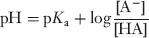# Henderson-Hasselbalch equation

Henderson-Hasselbalch equation
Hen·der·son-Has·sel·balch equation 'hen-dər-sən-'has-əl-.bälk- n an equation that equates the pH of a buffered solution (as the blood) to the sum of the cologarithm (p) of the dissociation constant (K) of the acid in the buffer and the logarithm of the ratio of the concentration ([ ]) of the salt to that of the acid and that is written
Henderson Lawrence Joseph (1878-1942)
American biochemist. Henderson is remembered for his discovery of the chemical means by which acid-base equilibria are maintained in nature. In his investigations of body fluids, he discovered that the formation of carbonic acid from carbon dioxide and water in the presence of the salt of the acid (bicarbonates) is the only system in nature that maintains acid-base equilibrium. Henderson developed a chemical equation used to describe these systems, known as physiological buffers. This equation is of fundamental importance to biochemistry.
Hasselbalch Karl Albert (1874-1962)
Danish biochemist. In 1916 Hasselbalch converted the equation developed by Lawrence Henderson into logarithmic form. Although the Henderson-Hasselbalch equation is only approximately true, it still remains the most useful mathematical model for treating problems dealing with buffer solutions.

* * *

an equation giving the pH of a buffer system:where [HA] is the concentration of the free acid, [A] is the concentration of the ionized form, and pKa> is the negative logarithm of the acid dissociation constant (Ka>).

Medical dictionary. 2011.

### Look at other dictionaries:

• Henderson–Hasselbalch equation — In chemistry, the Henderson–Hasselbalch (often misspelled as Henderson–Hasselbach ) equation describes the derivation of pH as a measure of acidity (using pKa, the acid dissociation constant) in biological and chemical systems. The equation is… …   Wikipedia

• Ecuación de Henderson-Hasselbalch — Saltar a navegación, búsqueda La ecuación de Henderson Hasselbalch (frecuentemente mal escrito como Henderson Hasselbach) fórmula bioquímica que se utiliza para calcular el pH, de una solución buffer, o tampón, a partir del pKa (la constante de… …   Wikipedia Español

• Équation de Henderson-Hasselbalch — En chimie, l équation de Henderson Hasselbach est une équation donnant le pH d un système tamponné. Elle est utilisée dans le domaine de la médecine pour déterminer le pH sanguin à partir des concentrations en ion bicarbonate et en acide… …   Wikipédia en Français

• Équation de Henderson-Hasselbach — Équation de Henderson Hasselbalch En chimie, l équation de Henderson Hasselbalch (également appelée équation de Henderson Hasselbach) est une équation donnant le pH d un système tamponné. Elle est utilisée dans le domaine de la médecine pour… …   Wikipédia en Français

• equation — A statement expressing the equality of two things, usually with the use of mathematical or chemical symbols. [L. aequare, to make equal] alveolar gas e. the e. defining the steady state relation of the alveolar oxygen pressure to the barometric… …   Medical dictionary

• Hasselbalch — Karl, Danish biochemist and physician, 1874–1962. See Henderson H. equation …   Medical dictionary

• Henderson — Lawrence J., U.S. biochemist, 1878–1942. See H. Hasselbalch equation …   Medical dictionary

• Charlot equation — The Charlot equation, named after Gaston Charlot, is used in analytical chemistry to relate the hydrogen ion concentration, and therefore the pH, with the formal analytical concentration of an acid and its conjugate base. It can be used for… …   Wikipedia

• Karl Albert Hasselbalch — (1 November 1874, Aastrup, Denmark 19 September 1962) was a physician and chemist. He was a pioneer in the use of pH measurement in medicine (with Christian Bohr, father of Nils Bohr), and he described how the affinity of blood for oxygen was… …   Wikipedia

• Lawrence Joseph Henderson — Infobox Scientist name = Lawrence Joseph Henderson box width = image width =150px caption = Lawrence Joseph Henderson birth date = June 3, 1878 birth place = Lynn, Massachusetts death date = February 10, 1942 death place = Cambridge,… …   Wikipedia